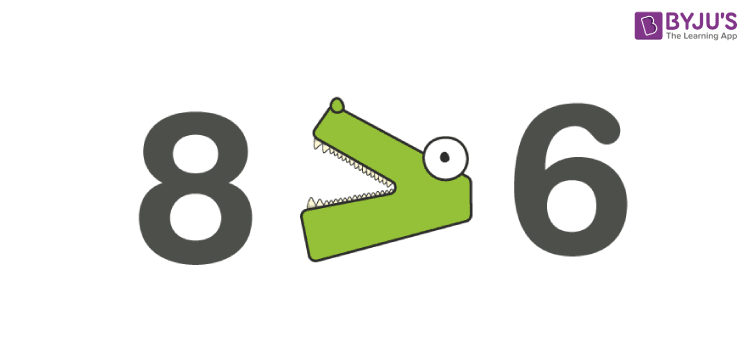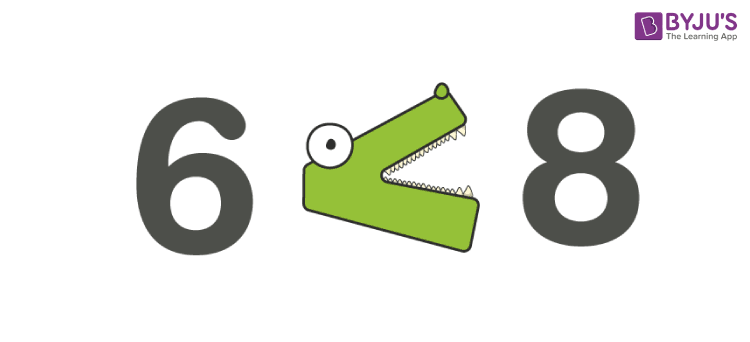# Greater than Symbol

In mathematics, the greater than symbol is a basic mathematical symbol which is used to represent the inequality between two values. The symbol used to represent the greater than inequality is “ > “. This is the universally adopted math symbol of two equal length strokes joining in the acute angle at the right. Also, learn less than symbol, which denotes just the opposite of greater than expression.

The typical use of the greater than symbol is to compare two values in which the first number is greater than the second number or one value is greater than the other one. The greater than symbol is an approximation of a closing angle bracket. We can see its huge application in descending order, where the arrangement from the largest number to smallest number is done using greater than a symbol.

## Greater than Symbol Example

• 5 > 2: 5 is greater than 2
• 1.2 > 0.8: 1.2 is greater than 0.8
• -0.1 > -0. 6: -0.1 is greater than -0.6

Here the list of all the symbols along with greater than symbol with its definition and examples are given in detail.

 Symbol Description Example = Equal to 3+2 = 5 ≠ Not equal to 4 + 2 ≠ 5 > Greater than 6 > 4 ≥ Greater than or equal to Students ≥ 5 < Less than 3 < 5 ≤ Less than or equal to Students ≤ 50

## How to Use Greater than Symbol?

The best way to remember the greater than symbol is the alligator method. When you compare two values, think of the alligator method. We know that the alligator’s mouth is always pointed to the largest value as it can eat as much as possible. In order to represent the greater value by using greater than symbol the alligator mouth always opens to the left.

 Related Links Fractions Mixed Fractions Decimals Integers

## Greater than And Less than Symbol

To represent the greater value by comparison with other numbers, the alligator’s mouth is always towards the larger value. To represent the inequality between two numbers say 6 and 8, it is written in two different ways as given below.

### Greater than Sign

• 8 > 6 (or)### Less than Sign

The above expression can also be represented using less than sign as:

• 6 < 8Both give the same meaning as 8 is greater than 6.

There are some other terminologies used alternately with greater than such as more than, as much as, as old as, not less than, or at least.

### Greater Than or Equal to Sign

Sometimes we may observe scenarios where the result obtained by solving an expression  for a variable, which are greater than or equal to each other. In such cases, we can use the greater than or equal to symbol, i.e. ≥. For example, x ≥ -3 is the solution of a certain expression in variable x.

## Word Problems

Before solving the greater than symbol inequality word problems, make attention to some strategies given below to solve the problem

• Go through the entire problem
• Highlight the important keywords to solve the problem
• Recognize the variables
• Write down the equation
• Solve the problem
• Write the solution in a complete sentence format

Question: Ramya has 500 rupees in a savings account at the beginning of the summer. She wants to have at least rupees 200 in the account by the end of the summer. She withdraws rupees 25 every week. Write the inequality expression that represents the Ramya situation.

Solution:

• Highlight the important keyword given

The keyword given here is at least

• Assume the variable

Let x be the number of weeks

• So, the inequality expression is

500 – 25x ≥ 200

Note that here we used greater than or equal symbol instead of using greater than a symbol because Ramya wants to have money which is equal to greater than 200 by the end of the summer. So, greater than or equal to the inequality symbol is used.

### Examples

Example 1: Fill in the blanks with the suitable sign.

1. 45 ____ 84
2. 77 ____ 76
3. 12 + 27 ____ 39
4. 98 – 23 ____ 54

Solution:

1. 45 < 84
2. 77 > 76
3. 12 + 27 = 39
4. 98 – 23 ____ 54

Here, 98 – 23 = 75 > 54

Thus, 98 – 23 > 54

Example 2: In the following pair of numbers, identify the greater number and write using the symbol.

-18, -25; -1.5, -1.8

Solution:

Given numbers are: -18, -25

In negative numbers, the number with greater numeral value is less than the number with lesser numerical value.

Thus, -18 is greater than -25, i.e. -18 > -25.

Register with BYJU’S – The Learning App for some important maths-related articles and also watch videos to learn with ease.

## Frequently Asked Questions – FAQs

Q1

### What do you mean by greater than symbol?

Greater than symbol is used when we have to compare two values, in which one value is greater than another value. It is denoted by the symbol ‘>’. Examples are:
10>9, 10 is greater than 9 which is true
7>1, 7 is greater than 1
5>2, 5 is greater than 2
Q2

### What do you mean by less than symbol?

Less than symbol is used to compare two quantities where one is smaller than the other. It is denoted by the symbol ‘<’. Examples are:
9<10, 9 is less than 10
1<7, 1 is less than 7
2<5, 2 is less than 5
Q3

### What is smaller than the symbol?

The smaller than symbol is, the less than a symbol, used to compare if one value is smaller than the other one. For example, 8 is smaller than 10, 8<10.
Q4

### What is greater than or equal to a symbol?

In linear inequalities, greater than or equal to the symbol is used when we are uncertain if the one value is greater than or equal to another value. It is represented by the symbol ‘≥’. In the same way, less than or equal to a symbol (≤) is used to represent when one quantity is either smaller than or equal to another quantity.
Example: 4x + 7 ≥ 6x +3
Q5

### Which is greater 0.5 or 0.25?

0.5 is greater than 0.25. Symbolically it is represented as 0.5>0.25.
Q6

### Which is greater ⅗ or ½?

We can check either by rationalising the denominator or finding the actual value of ⅗ and ½.
By rationalisation, we get;
⅗ x (2/2) and ½ x (5/5)
6/10 and 5/10
So as we can see denominators are equal and 6 is greater than 5, therefore,
⅗ > ½
Check:
⅗ = 0.6 and ½ = 0.5
0.6>0.5
Test your knowledge on Greater Than Symbol